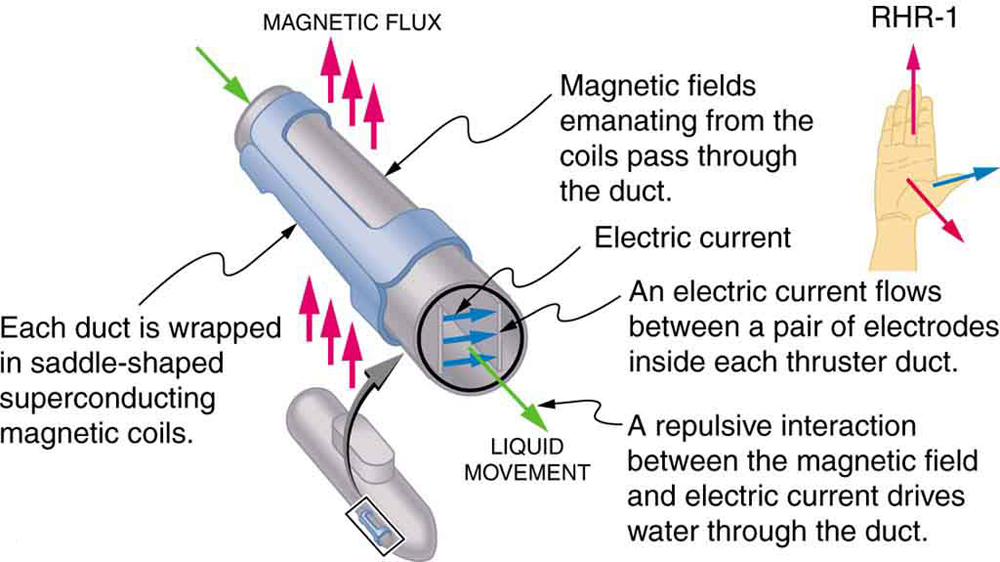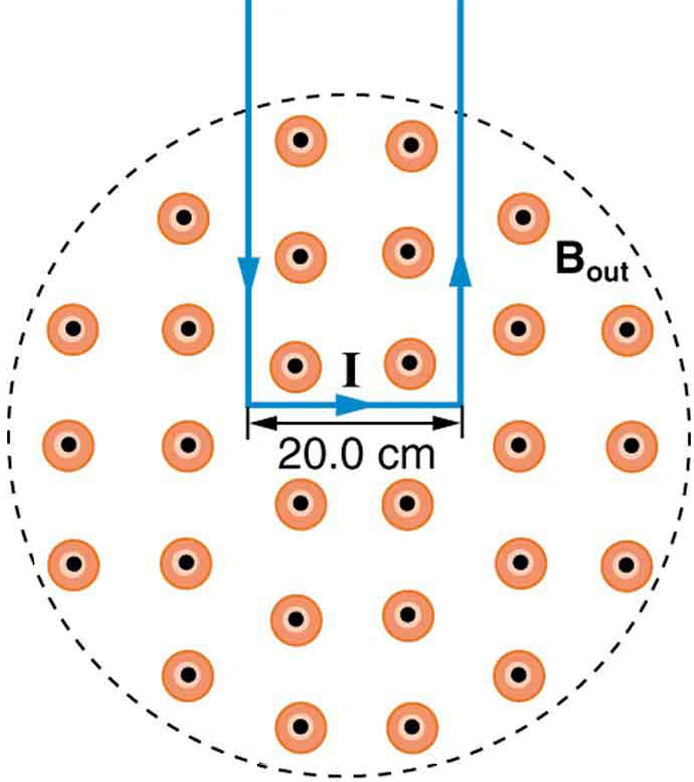# 22.7 Magnetic force on a current-carrying conductor  (Page 2/2)

 Page 2 / 2An MHD propulsion system in a nuclear submarine could produce significantly less turbulence than propellers and allow it to run more silently. The development of a silent drive submarine was dramatized in the book and the film The Hunt for Red October .

## Section summary

• The magnetic force on current-carrying conductors is given by
$F=\text{IlB}\phantom{\rule{0.25em}{0ex}}\text{sin}\phantom{\rule{0.25em}{0ex}}\mathrm{\theta ,}$
where $I$ is the current, $l$ is the length of a straight conductor in a uniform magnetic field $B$ , and $\theta$ is the angle between $I$ and $B$ . The force follows RHR-1 with the thumb in the direction of $I$ .

## Conceptual questions

Draw a sketch of the situation in [link] showing the direction of electrons carrying the current, and use RHR-1 to verify the direction of the force on the wire.

Verify that the direction of the force in an MHD drive, such as that in [link] , does not depend on the sign of the charges carrying the current across the fluid.

Why would a magnetohydrodynamic drive work better in ocean water than in fresh water? Also, why would superconducting magnets be desirable?

Which is more likely to interfere with compass readings, AC current in your refrigerator or DC current when you start your car? Explain.

## Problems&Exercises

What is the direction of the magnetic force on the current in each of the six cases in [link] ?

(a) west (left)

(b) into page

(c) north (up)

(d) no force

(e) east (right)

(f) south (down)

What is the direction of a current that experiences the magnetic force shown in each of the three cases in [link] , assuming the current runs perpendicular to $B$ ?

What is the direction of the magnetic field that produces the magnetic force shown on the currents in each of the three cases in [link] , assuming $\mathbf{\text{B}}$ is perpendicular to $\mathbf{\text{I}}$ ?

(a) into page

(b) west (left)

(c) out of page

(a) What is the force per meter on a lightning bolt at the equator that carries 20,000 A perpendicular to the Earth’s $3\text{.}\text{00}×{\text{10}}^{-5}\text{-T}$ field? (b) What is the direction of the force if the current is straight up and the Earth’s field direction is due north, parallel to the ground?

(a) A DC power line for a light-rail system carries 1000 A at an angle of $\text{30.0º}$ to the Earth’s $\text{5.00}×{\text{10}}^{-5}\phantom{\rule{0.25em}{0ex}}\text{-T}$ field. What is the force on a 100-m section of this line? (b) Discuss practical concerns this presents, if any.

(a) 2.50 N

(b) This is about half a pound of force per 100 m of wire, which is much less than the weight of the wire itself. Therefore, it does not cause any special concerns.

What force is exerted on the water in an MHD drive utilizing a 25.0-cm-diameter tube, if 100-A current is passed across the tube that is perpendicular to a 2.00-T magnetic field? (The relatively small size of this force indicates the need for very large currents and magnetic fields to make practical MHD drives.)

A wire carrying a 30.0-A current passes between the poles of a strong magnet that is perpendicular to its field and experiences a 2.16-N force on the 4.00 cm of wire in the field. What is the average field strength?

1.80 T

(a) A 0.750-m-long section of cable carrying current to a car starter motor makes an angle of $\text{60º}$ with the Earth’s $5\text{.}\text{50}×{\text{10}}^{-5}\phantom{\rule{0.25em}{0ex}}\text{T}$ field. What is the current when the wire experiences a force of $\text{7.00}×{\text{10}}^{-3}\phantom{\rule{0.25em}{0ex}}N$ ? (b) If you run the wire between the poles of a strong horseshoe magnet, subjecting 5.00 cm of it to a 1.75-T field, what force is exerted on this segment of wire?

(a) What is the angle between a wire carrying an 8.00-A current and the 1.20-T field it is in if 50.0 cm of the wire experiences a magnetic force of 2.40 N? (b) What is the force on the wire if it is rotated to make an angle of $\text{90º}$ with the field?

(a) $\text{30º}$

(b) 4.80 N

The force on the rectangular loop of wire in the magnetic field in [link] can be used to measure field strength. The field is uniform, and the plane of the loop is perpendicular to the field. (a) What is the direction of the magnetic force on the loop? Justify the claim that the forces on the sides of the loop are equal and opposite, independent of how much of the loop is in the field and do not affect the net force on the loop. (b) If a current of 5.00 A is used, what is the force per tesla on the 20.0-cm-wide loop?A rectangular loop of wire carrying a current is perpendicular to a magnetic field. The field is uniform in the region shown and is zero outside that region.

discuss under damped
resistance of thermometer in relation to temperature
how
Bernard
that resistance is not measured yet, it may be probably in the next generation of scientists
Paul
Is fundamental quantities under physical quantities?
please I didn't not understand the concept of the physical therapy
physiotherapy - it's a practice of exercising for healthy living.
Paul
what chapter is this?
Anderson
this is not in this book, it's from other experiences.
Paul
Sure
What is Boyce law
how to convert meter per second to kilometers per hour
Divide with 3.6
Mateo
multiply by (km/1000m) x (3600 s/h) -> 3.6
2 how heat loss is prevented in a vacuum flask
what is science
Helen
logical reasoning for a particular phenomenon.
Ajay
I don't know anything about it 😔. I'm sorry, please forgive 😔
due to non in contact mean no conduction and no convection bec of non conducting base and walls and also their is a grape between the layer like to take the example of thermo flask
Abdul
dimensions v²=u²+2at
what if time is not given in finding the average velocity?
the magnetic circuit of a certain of the flux paths in each of the long and short sides being 25cm and 20cm reprectielectrove. there is an air gap of 2mm long in one the long sides if a flux density of 0.8weber/m is to produce in the magnet of 1500 turns..
How do you calculate precision
what module is that?
Fillemon
Chemisty 1A?
Fillemon
No it has something to do with measurements bro... What we did today in class
Sacky
Tah bra honestly I didn't understand a thing in that class..when re your Tutorials?
Fillemon
Friday bro... But the topics we did are in this app... Just try to master them quickly before the test dates... Are you done with the Maths sheet
Sacky
I eat ass
Anderson
I'll work on the maths sheet tomorrow bra @Sacky Malyenge but I'll try mastering them
Fillemon
I'll eat your mom's ass with a side of tendies
Anderson
@Fillemon Nanwaapo
Anderson
lol, hush
Emi
There are very large numbers of charged particles in most objects. Why, then, don’t most objects exhibit static electricity?
Because there's an equal number of negative and positive charges... objects are neutral in nature
NELSON
when a ball rolls on a smooth level ground,the motion of its centre is?
what is electro magnetic field?
Mary
electromagnetic field is a special type of field been produced by electric charges..!!! like the word electro from Electricity and the word magnetic from Magnetism.. so it is more of a join field..!!!
NELSON
Electromagnetic field is caused by moving electric charge
when a ball rolls on a smooth level ground,the motion of its centre is?
Mumeh
what's the relationship btw displacement and position
displacement is the change of position 8======✊=D 💦💦
AndersonByBy Anindyo MukhopadhyayByByBy